plot_model creates a tibble with up to 4 individual plots and one combined plot (all ggplot2 objects) for each IND~pressure pair in the input tibble. The number of plots generated depends on the information provided in the input tibble. If all model IND~pressure modeling functions have been applied to create the final input tibble all five plots will be produced.

plot_model(
init_tbl,
mod_tbl,
choose_thresh_gam = NULL,
pos_label = "topleft",
)

## Arguments

init_tbl The output tibble of the ind_init function. Any output tibble from the IND~pressure modeling functions. Selects the threshold_GAM for the thresh_plot, which is relevant if several models are listed in thresh_models. The default is NULL, which shows the best performing threshold_GAM (based on the GCV as selection criterion). Specifies the position of the annotation in the plot. Should be one of "topleft" (default), "topright", "bottomleft" or "bottomright". For more details see place_text. logical; if TRUE, each plot will have a header including the IND name, pressure name(s) and the model type.

## Value

The function returns a tibble, including the following elements:

id

Numerical IDs of the IND~press combinations.

ind

Indicator names.

press

Pressure names.

response_plot

A list-column of ggplot2 objects that show the observed (black points) and predicted IND response to the single pressure (based on the training data). The solid blue line represents the predicted mean and the transparent polygon the 95% confidence interval. The effective degrees of freedom (edf), R_sq, and p-value from the fitted model are additionally provided. The input needed for this plot is generated from the model_gam or model_gamm functions.

predict_plot

A list-column of ggplot2 objects that show the robustness of the modeled relationship expressed as the predictive performance (the NRMSE) on a test dataset, e.g the last years of the time series. The solid green line represents the predicted IND value given the observed pressure value for that particular year (both in the training and test data, the latter displayed as green triangles). The transparent polygon represents the 95% confidence interval. Observed IND values of the test data are shown as black triangle, the trainings observations are presented as black circles. The input needed for this plot is generated from the model_gam or model_gamm functions.

deriv_plot

A list-column of ggplot2 objects that show the first derivatives (S) of non-linear IND~pressure response curves (edf > 1.5) and the proportion of the pressure range where the IND shows no further significant change (i.e., slope approximates zero). Black triangles represent values at the pressures boundary where the zero line falls into the confidence interval, which indicates no further significant IND change. Circle represent values that were considered positive for the calculation of the pressure range (see for more details calc_deriv). The input needed for this plot is generated from the calc_deriv function.

thresh_plot

A list-column of ggplot2 objects that show the observed IND response curve for a specific pressure under a low (left panel, in black) and high (right panel, in red) regime of an interacting 2nd pressure variable. The solid lines represent the predicted mean and the transparent polygons the 95% confidence intervals. Filled circles represent the observed training observations in each regime. If no thresh_plot is created for that IND~pressure pair, no interaction was found. If more than one interacting pressure variable has been detected, i.e. more than one threshold-GAM performed better than its corresponding GAM, the threshold-GAM with the best GCV will be displayed. The input needed for this plot is generated from the test_interaction function. If the plot shows strange patterns such as smoothers hardly differ in both regimes with wide confidence intervals at the edge or few data points in one regime check the model diagnostics of this threshold model! Outliers can cause such patterns or if threshold is at the edge of the pressure range or other thresholds are similarly likely (see also plot_diagnostics.)

all_plots

A list-column of ggplot2 objects that show all plots together using additional drawing canvas from the cowplot package on top of ggplot2.

Other IND~pressure modeling functions: find_id(), ind_init(), model_gamm(), model_gam(), plot_diagnostics(), scoring(), select_model(), test_interaction()

## Examples

# \donttest{
# Using some models of the Baltic Sea demo data in this package
mod_tbl <- all_results_ex[4:5, ]
init_tbl <- ind_init_ex[4:5, ]
dat <- plot_model(init_tbl, mod_tbl, pos_label = "topleft")
#> Warning: Removed 1 row(s) containing missing values (geom_path).#> Warning: Removed 1 rows containing missing values (geom_point).#> Warning: Removed 1 row(s) containing missing values (geom_path).#> Warning: Removed 1 rows containing missing values (geom_point).dat$response_plot[]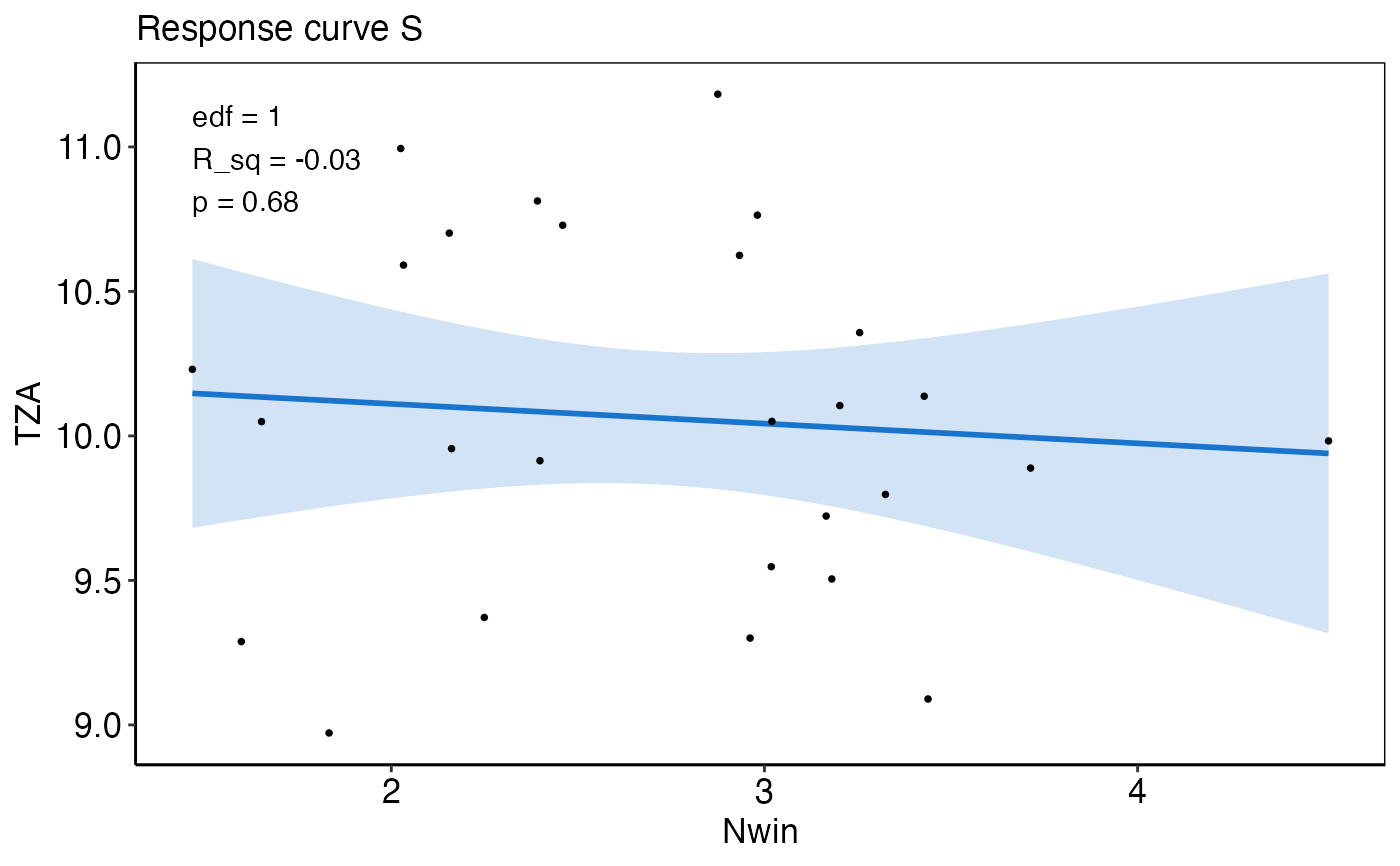dat$predict_plot[]
#> Warning: Removed 1 row(s) containing missing values (geom_path).#> Warning: Removed 1 rows containing missing values (geom_point).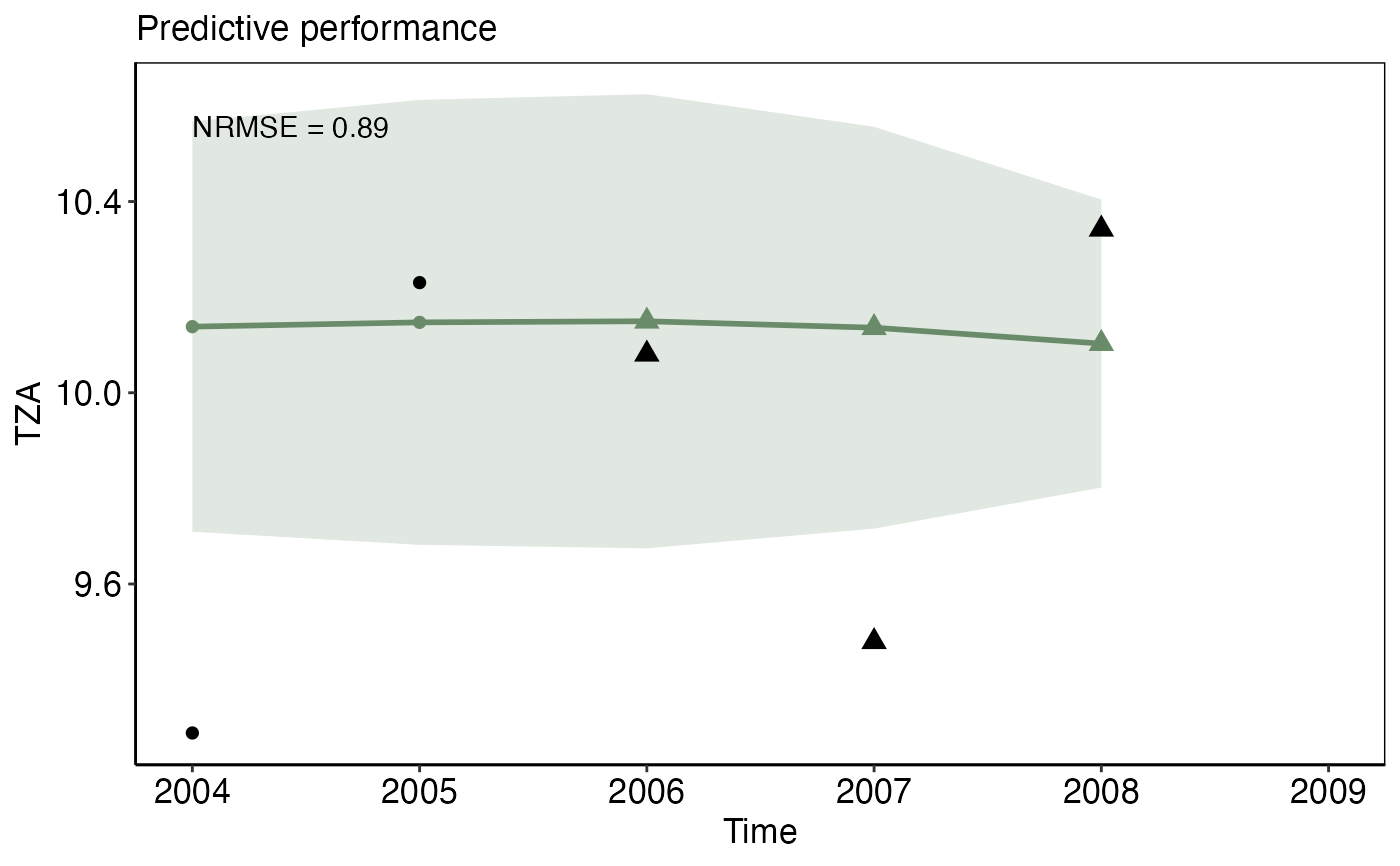dat$deriv_plot[]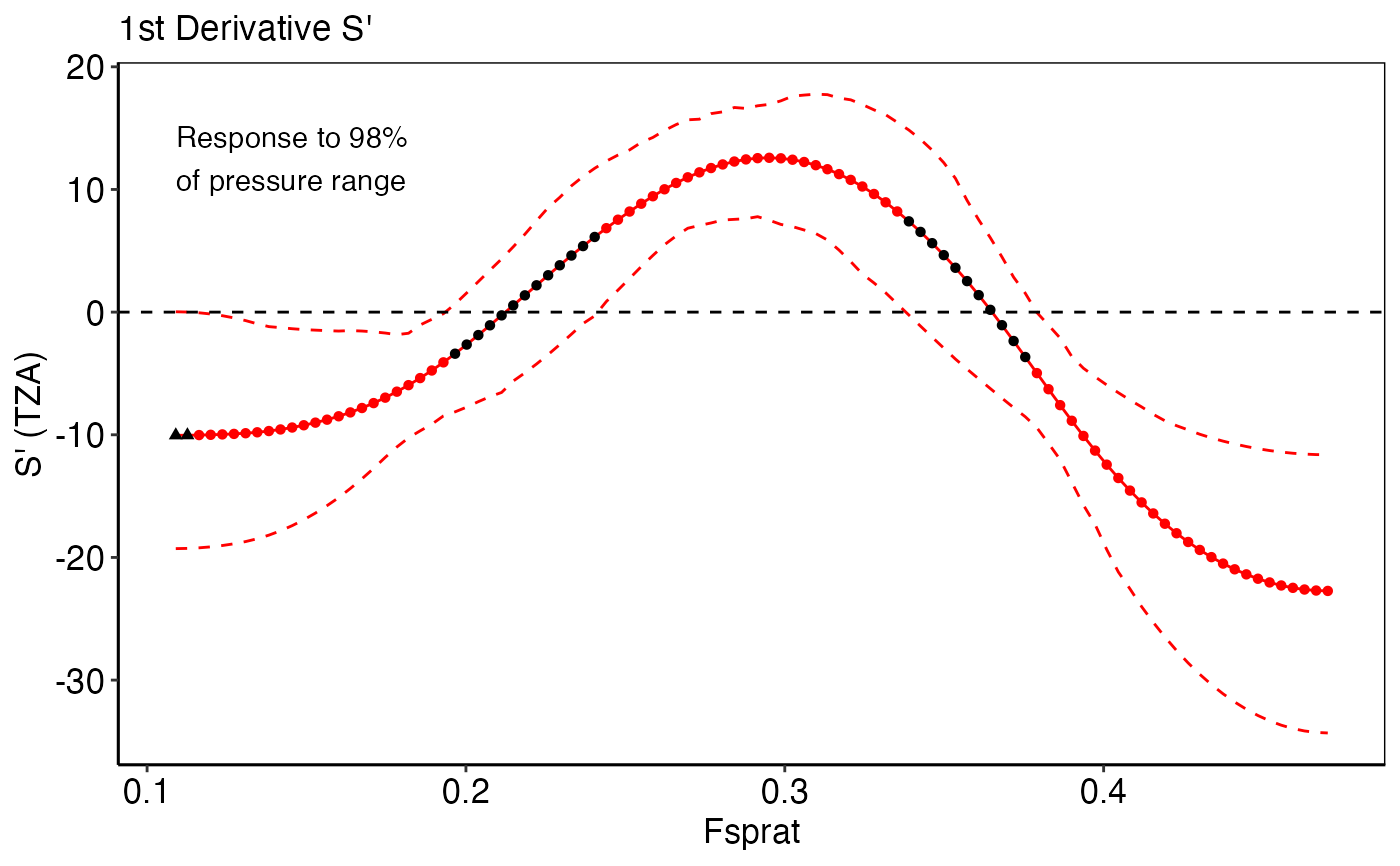dat$thresh_plot[]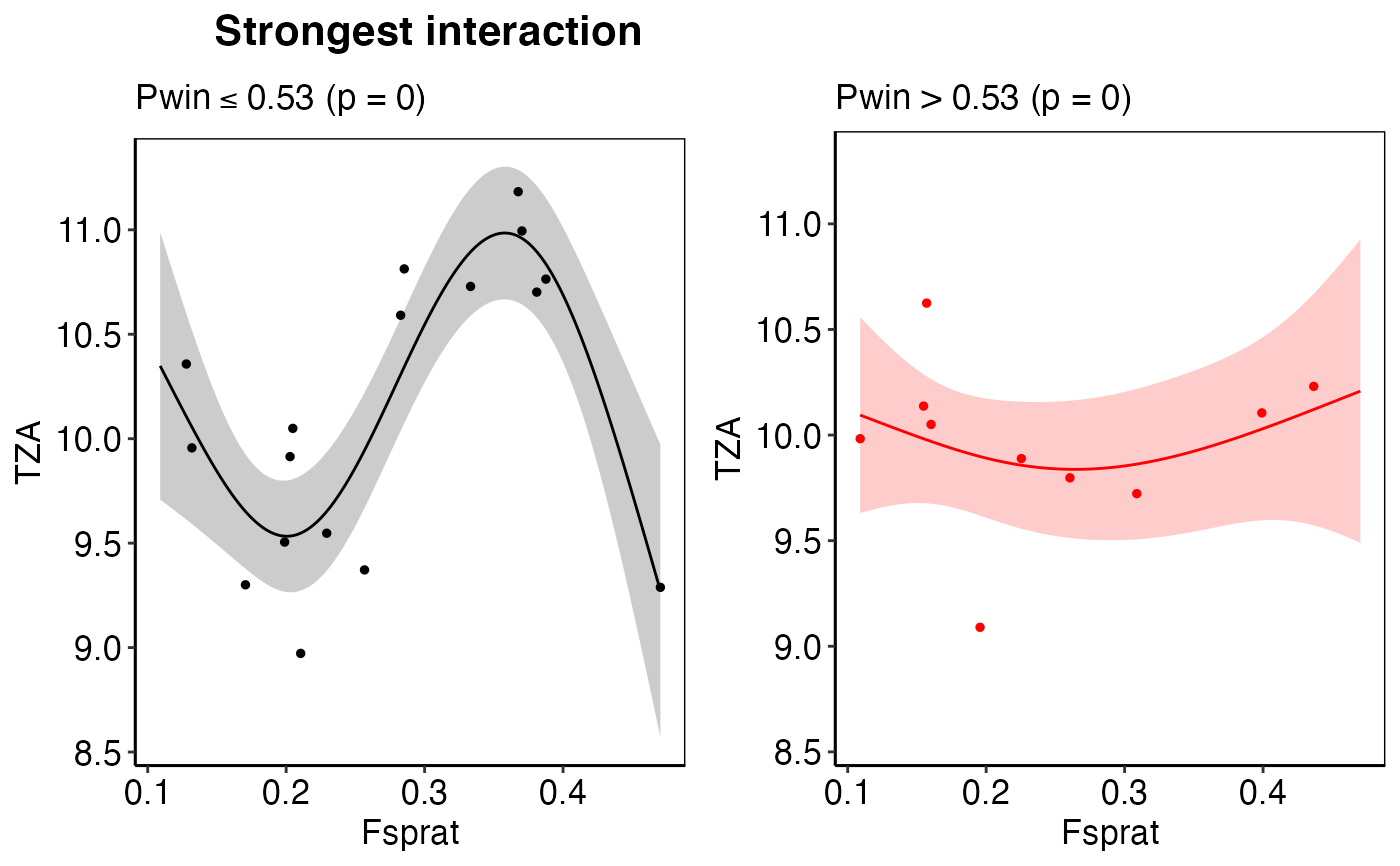dat$all_plots[]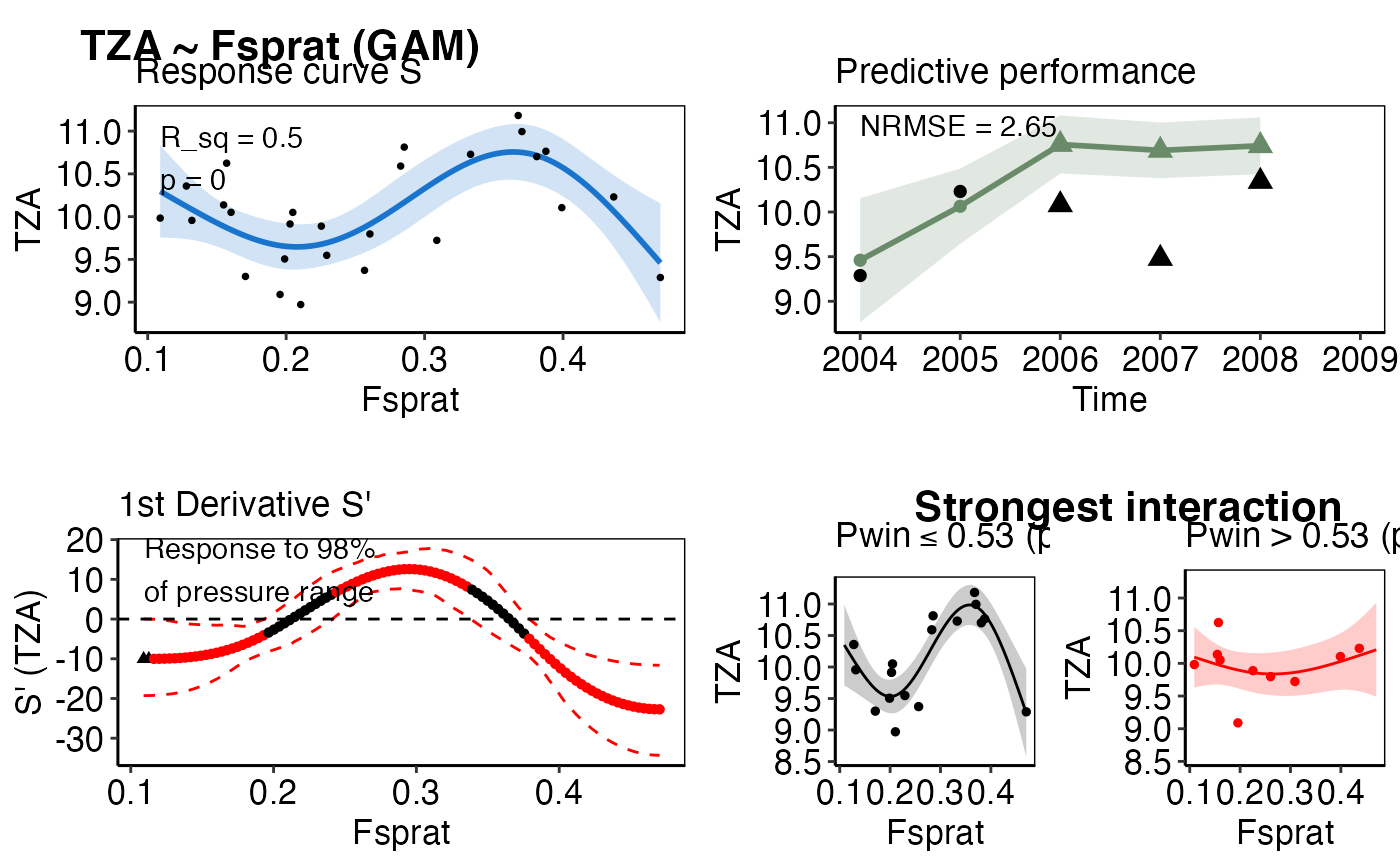# Apply function to all sign. models and save specific plots id <- which(all_results_ex$p_val <= 0.05)
init_tbl <- ind_init_ex[id, ]
mod_tbl <- all_results_ex[id, ]
dat <- plot_model(init_tbl, mod_tbl, pos_label = "bottomright")
#> Warning: Removed 1 row(s) containing missing values (geom_path).#> Warning: Removed 1 rows containing missing values (geom_point).#> Warning: Removed 1 row(s) containing missing values (geom_path).#> Warning: Removed 1 rows containing missing values (geom_point).#> Warning: Removed 1 row(s) containing missing values (geom_path).#> Warning: Removed 1 rows containing missing values (geom_point).#> Warning: Removed 1 row(s) containing missing values (geom_path).#> Warning: Removed 1 rows containing missing values (geom_point).#> Warning: Removed 1 row(s) containing missing values (geom_path).#> Warning: Removed 1 rows containing missing values (geom_point).#> Warning: Removed 1 row(s) containing missing values (geom_path).#> Warning: Removed 1 rows containing missing values (geom_point).#> Warning: Removed 1 row(s) containing missing values (geom_path).#> Warning: Removed 1 rows containing missing values (geom_point).#> Warning: Removed 1 row(s) containing missing values (geom_path).#> Warning: Removed 1 rows containing missing values (geom_point).#> Warning: Removed 1 row(s) containing missing values (geom_path).#> Warning: Removed 1 rows containing missing values (geom_point).#> Warning: Removed 1 row(s) containing missing values (geom_path).#> Warning: Removed 1 rows containing missing values (geom_point).#> Warning: Removed 1 row(s) containing missing values (geom_path).#> Warning: Removed 1 rows containing missing values (geom_point).#> Warning: Removed 8 rows containing missing values (geom_point).#> Warning: Removed 1 row(s) containing missing values (geom_path).#> Warning: Removed 1 rows containing missing values (geom_point).#> Warning: Removed 1 row(s) containing missing values (geom_path).#> Warning: Removed 1 rows containing missing values (geom_point). pdf(file.path(tempdir(), "Plot.pdf"), height=10, width=10)
dat\$all_plots
#> []#>
#> []#>
#> []#>
#> []#>
#> []#>
#> []#>
#> []#>
#> []#>
#> []#>
#> []#>
#> []#>
#> []#>
#> []#>  dev.off()
#> agg_png
#>       2 # }